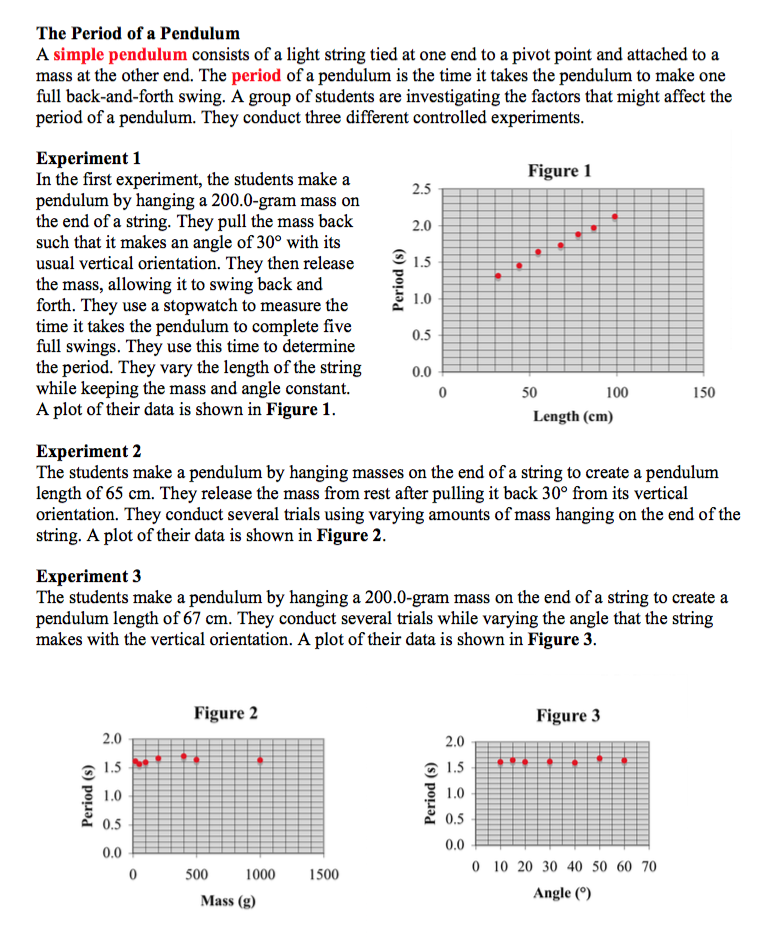###Period of a Pendulum

Resource:
The Science Reasoning Center: Period of a Pendulum

Description:
This passage describes three simple experiments conducted by students in order to determine the variables that affect the period of a pendulum. Each experiment is described and the results are presented in the form of a graph. Questions target a student's ability to understand the design of an experiment, to identify the effect of one variable upon another variable, to draw a conclusion that is consistent with collected data, to read values off a graph, to extrapolate beyond the range of values on a graph, and to use provided data to make a prediction about the period that would result under a given set of conditions.

This activity aligns with the three dimensions of the Next Generation Science Standards in the manner described below:

Crosscutting Concepts
 Scale, Proportion, and Quantity:  Algebraic thinking is used to examine scientific data and predict the effect of a change in one variable on another (e.g., linear growth vs. exponential growth). Exploring factors that affect the period of a pendulum is a relatively simple experiment that students can design and carry out.  This passage describes such an experiment and provides the reader with typical data.  As students consider three mini-experiments and the data collected, they are asked to predict the effect of changing one variable (length of string, mass, or angle of release) on another (pendulum period).   One valuable outcome of this analysis is that students realize that not every factor tested actually affects a pendulum’s period. Cause and Effect:  Systems can be designed to cause a desired effect. At the close of this passage students are presented with several different pendula—each with a unique mass, length of string, and release angle.  Students are then asked, “Which pendulum would have the largest period?”  Identifying the pendulum that meets this criterion requires students to carefully analyze results from each of three experiments.  Such analysis allows students to see how each factor affects a pendulum’s period in order to identify the one that meets the requested criterion.

Practices
 Analyzing and Interpreting Data: Apply concepts of statistics and probability (including determining function fits to data, slope, intercept, and correlation coefficient for linear fits) to scientific and engineering questions and problems, using digital tools when feasible. Experimental results often require scientists to discern whether small fluctuations in data are significant or merely the result of error in measurement.  This passage provides the student with such experimental results.  Students must apply basic principles of statistics and probability to discern whether mass and/or release angle affect a pendulum’s period.  As they do, students may be surprised by these results. Constructing Explanations and Designing Solutions:  Apply scientific principles and evidence to provide an explanation of phenomena and solve design problems, taking into account possible unanticipated effects. End-of-passage questions present students with several higher level questions that require the synthesis of multiple data presentations.  In one such question, length, mass, and release angle are given for a ‘new’ pendulum and students are asked to explain if it is possible (and why) to use the data provided in these experiments to estimate the period of this ‘new’ pendulum.  Such application of experimental evidence also forces students to take into account the perhaps unanticipated effects that not every variable impacts a pendulum’s period. Planning and Carrying Out Investigations:  Plan an investigation or test a design individually and collaboratively to produce data to serve as the basis for evidence in building/revising models, supporting explanations for phenomena, or testing solutions to problems. Consider possible confounding variables and ensure variables are controlled. While students read about a set of simple experiments that have already been performed, they are faced with several end-of-passage questions that require them to analyze why the experimenter designed the investigation in the way that it was done.  Students are asked to identify the goal of each experiment, the purpose of timing over multiple swings, and dependent/independent variables.  For one experiment, students are asked to identify possible confounding variables and how the experimenter ensured that variables were controlled.

Associated Reading from The Physics Classroom

View Infographic.
(Coming Soon)

### Search the NGSS Corner

Maybe you're looking for something really specific that pertains to a desired topic and emphasizes one or more of the listed NGSS dimensions. Why not try a search of this section of our website? Simply select from one or more of the pull-down menus and click Search  This page will reload and a collection  of possibilities will be displayed in this section of the page and sorted by relevancy.